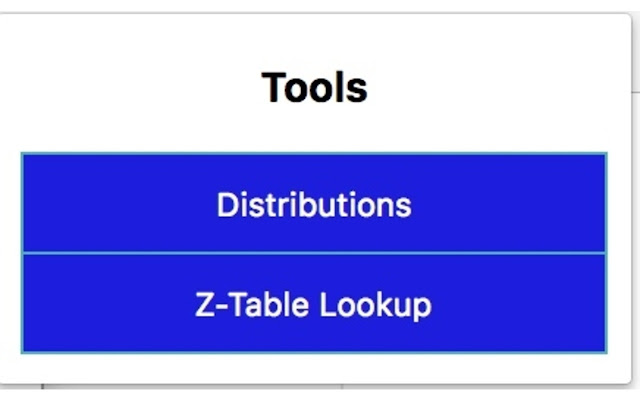# Probability Toolkit

Description from extension meta

## A tool to help quickly calculate different probability values for various distributions.

Image from storeDescription from store Calculate different probability values, expected value, and variance for the following distributions: Binomial, Geometric, Hypergeometric, Poisson, Negative Binomial, Uniform, Normal, Exponential, Chi-square, and Gamma. Moreover, this tool offers quick z-table lookups for arbitrary ranges.

### Latest reviews

• (2019-12-31) Chantal Lee: Super useful for my statistics university course. I would highly recommend this extension!
• (2018-08-12) William Gan: It was a useful Extension for solving problems

Installs
146 history
Category
Rating